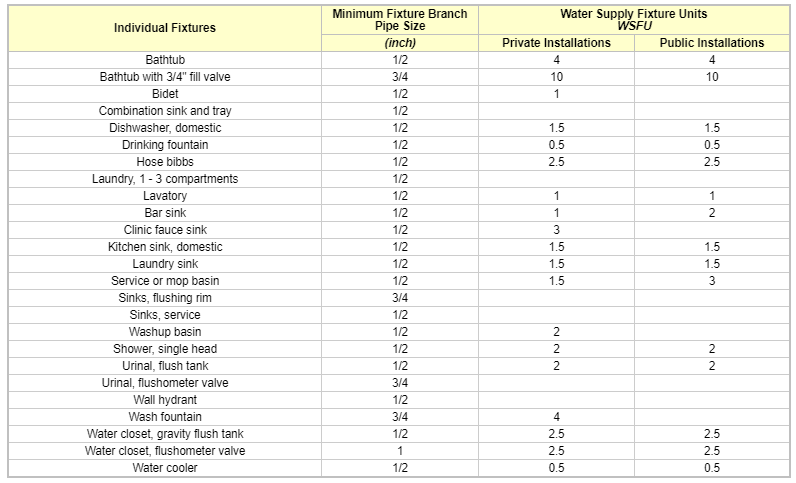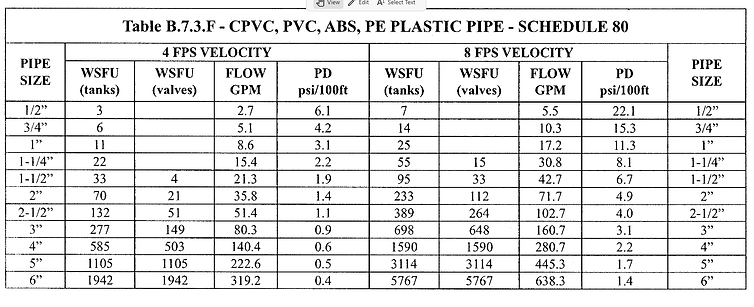top of page
##### Water Supply Calculations

Proper design of water-distribution system in a building is necessary to avoid excessive installed cost and in order that the various fixtures may function properly under normal conditions. The correct design results in piping, water heating, the storage facilities of sufficient capacity to meet the probable peak demand without wasteful excess in either piping or maintenance cost.

First step is to locate the source of water supply. We have two filling lines. One is the Ministry filling line size 1” and other is tanker filling line size 2”.

Minimum pressure required at water outlets

Minimum pressure required at fixture outlets shall be 15 psi according to the following standard code from the NSPC below.We start our calculation using following four steps:

(1) Estimating Demand (Water Supply Fixture Units) - WSFU

(2) Calculating Pipe Sizes for the whole building (Cold and Hot Water System)
(3) Calculating Pipe Sizes for the whole building (Cold and Hot Water System)
(4) Calculating Friction Pressure Drops or losses in Cold and Hot Water Pipes

Step 1: Estimating Demand (Water Supply Fixture Unit) - WSFU

The WSFU (Water Supply Fixture Units) is defined by the Uniform Plumbing Code (UPC) and can be used to determine water supply to fixtures and their service systems.

Water Supply Fixture Units (WSFU) is the standard method for estimating the water demand for a building. This system assigns an arbitrary value called a WSFU to each fixture in a building, based on the amount of water required and the frequency of use.For example, a water closet (tank) is assigned a WSFU of 2.5 fixture units (FU) while a sink (lavatory) is assigned 1.0 FU for "other than dwelling units". These values are based on the International Plumbing Code Water Supply Fixture Unit table. The difference in fixture units is due to the fact that a toilet requires more water than a sink. The frequency of use between a private sink and a water closet would be the same, since a person will normally use the water closet and the sink within the same bathroom visit. A public water closet  has a WSFU value of 5.0. Even though the water closet (tank) is the same as the private water closet and uses the same amount of water, a public water closet has a higher WSFU value. The public water closet has a higher usage frequency, which increases the WSFU value.

The water supply fixture units are distinguished between cold, hot or both. If a plumbing line serves only the cold water side of a fixture, then the corresponding value should be used. For example, a main line may serve the both cold and hot water, but then a branch line may go to the hot water heater. The branch line would only use the hot water value.

Using tables B.5.2 from NSPC code, we summaries the WSFU design data for each fixtures used.

The water supply fixtures units (WSFU) fed by a pipe is determined by the number of each plumbing fixture that is connected to the pipe and the governing plumbing code. The plumbing code establishes the WSFU value for each fixture type. The piping layout determines the amount of each fixture type that is fed by each pipe.

Consider a  building design with  the following fixture quantities. And using table B.5.2, we have following table:Step 2: Converting demand in WSFU to GPM (Gallons per minute)

The next step is to convert the WSFU value to gallons per minute (GPM). This volumetric flow rate will help to determine the pressure drop and fluid velocity within the pipe in the next and final step. The conversion from WSFU to GPM will depend on whether or not the connected fixtures are predominantly flush valve type or tank type.

Flush Valve vs. Tank: This distinction is common of toilets. A tank type toilet uses the tank fluid elevation to forcefully flush the toilet waste through the waste system. After a tank toilet is flushed, a fill line is used to fill up the tank. The fill time is typically around 20 seconds. At a residence or where there is infrequent use, this fill time is acceptable. However, in a public space with frequent use, this fill time is not acceptable. A flush valve toilet is used in these situations. A flush valve type toilet does not have a tank to provide the pressure to force the waste into the waste system. Thus, a flush valve toilet will require a much higher minimum pressure.

A pipe that feeds predominantly flush valve type fixtures will have a greater volumetric flow rate requirement than a pipe that feeds predominantly tank type fixtures.

Using table B.5.4 for converting demand in WSFU to GPM.

We find that:

140 WSFU = 53 GPM (Flush Tank values)

160 WSFU = 57 GPM (Flush tank values)

Using Interpolation we find that,

159 WSFU = 56.8 GPM. Since 1 GPM = 3.785 L/m, therefore 56.8 GPM = 215 L/m

We find that we need 56.8 GPM (215 L/min) of water to feed this building.

Therefore, our booster pump flow rate shall be a minimum of 56.8 GPM (215 L/min)

Step 3: Calculating Pipe Size all building (Cold and Hot Water System)

Once the WSFU and the appropriate GPM conversion are determined, then the quick sizing table can be used to select the appropriate pipe size. The first step in using this table is to select the pipe material and pipe sub-type.

LIMITATIONS OF VELOCITY: It is generally recommended that a maximum velocity at maximum probable demand in supply piping be limited to 8 fps (refer to attached NSPC, page 237 - "B.6 - Limitation of Velocity".Using above table, we can size the CWS and HWS individual branch line for CPVC, PVC, ABS etc pipes. For all other pipes refer to general Table B.7.3. Refer to below schematic riser diagrams for sizing of water supply pipes using above tables.

Consider schematic for CWS. The first segment (Ground Floor), which only serves a lavatory. Since this line only serves a single fixture then you can use the fixture table, which indicates that the minimum pipe size as 3/8”. However, the pipe length is fairly long and according to the quick sizing table, there will be a large pressure drop. So a 1/2” pipe should be used for the long run. The individual connection to the fixture will be 3/8”.

Up to 7 WSFU pipe size will be 1/2", WSFU up to 14 pipe size shall be 3/4" and so on.

Step 4: Calculating Major and Minor losses

(Calculating Friction Pressure Drops or losses in Cold and Hot Water Pipes)

We draw a schematic riser diagram for the longest path from the roof tank to the last fixture on the ground floor.

Using table B.7.3.F (for pipe losses - major loss & table B.9.7.D for fitting loss (minor losses).Take section A-B as a sample calculation:Calculating Water Heater Capacity:

This method utilizes average hourly data in gallons per hour (GPH) for various type of buildings. To calculate using this table 14.6, we count the fixtures, multiply the number of fixtures by the gallons per hour (GPH) for the fixture of that particular type of building and then add them. Then we multiply this total by the simultaneous usage factor to get the maximum hourly demand for the system. The minimum recommended storage volume then is calculated by multiplying the total demand by the storage factor.Consider this building is a school (for example). Using table 14.6 (Hot Water Demand per Fixture for various building type)bottom of page